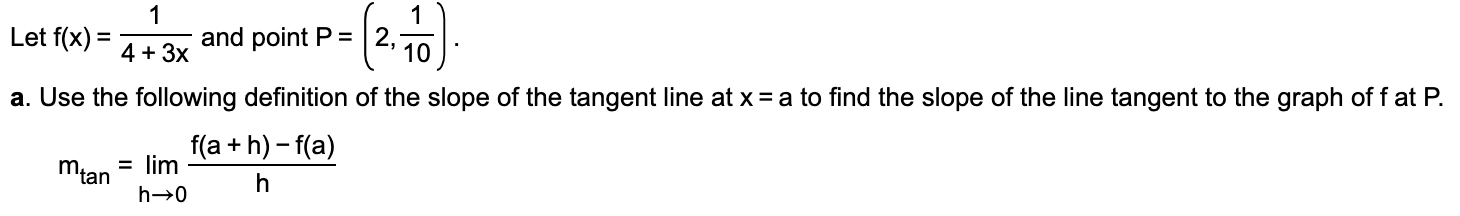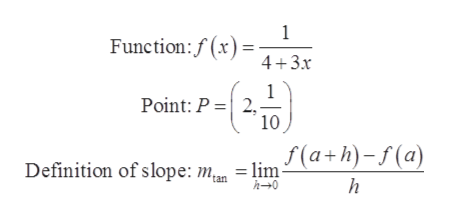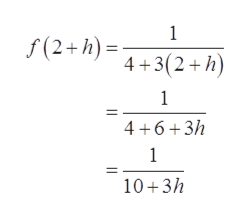11Let f(x) 43x and point P = | 2,7=10a. Use the following definition of the slope of the tangent line at x a to find the slope of the line tangent to the graph of f at P.f(a h) f(a)mtan limh-0h

Question

Hello,

I am struggling to find the slope of the tangent line for the attached problem.

Thanks.help_outlineImage Transcriptionclose1 1 Let f(x) 43x and point P = | 2,7 = 10 a. Use the following definition of the slope of the tangent line at x a to find the slope of the line tangent to the graph of f at P. f(a h) f(a) mtan lim h-0 h fullscreen
Step 1

To determine the slope of the tangent line using the definition of the slope at x = a.

Step 2

Given:help_outlineImage Transcriptionclose1 Function:f(x) 4+3x Point: P 2 10 Definition of slope: m.. = lim (a+h)-f(a) h 'tan h0 -19 fullscreen
Step 3

Calculate the slope of the tangent line at the point P = (2, 1/10).

Substitute 2 + ...help_outlineImage Transcriptionclose1 f(2+h) +3(2+h) 1 4 6 3/h 1 10 3h fullscreen

Want to see the full answer?

See Solution

Want to see this answer and more?

Our solutions are written by experts, many with advanced degrees, and available 24/7

See Solution
Tagged in

Limits